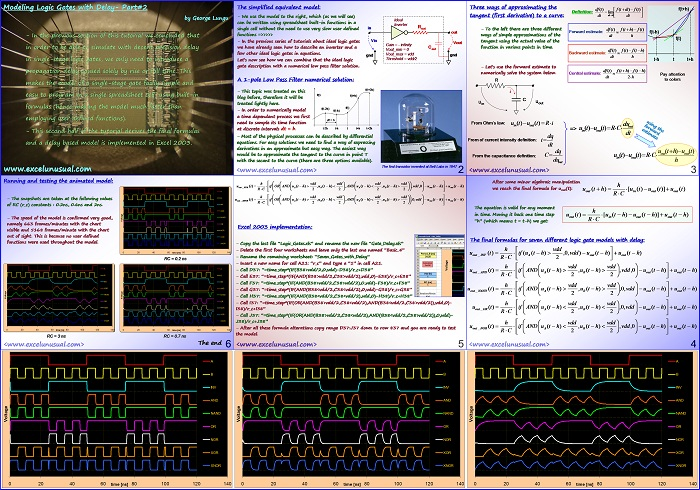# A Delay Based Animated Excel Model for Single-Stage Logic Gates – part#2

This is the second half of the delay based Excel model tutorial. In this part the final formulas are derived and implemented in Excel 2003.

The model proves to be fast and reliable and it is actually fairly easy to create. For maintaining good speed no user defined functions have been used here.## Modeling Logic Gates with Delay- Part#2

by George Lungu

– In the previous section of this tutorial we concluded that in order to be able to simulate with decent precision delay in single-stage logic gates, we only need to introduce a propagation delay caused solely by rise or fall time. This makes the model of a single-stage gate fairly simple and easy to program in a single spreadsheet cell, using built-in formulas (hence making the model much faster than employing user defined functions).

– This second half of the tutorial derives the final formulas and a delay based model is implemented in Excel 2003.

www.excelunusual.com

### The simplified equivalent model:

– We use the model to the right, which (as we will see) can be written using spreadsheet built-in functions in a single cell without the need to use (very slow) user in Rout defined functions =>>>>>

Ideal
Inverter
out

– In the previous series of tutorials about ideal logic gates we have already seen how to describe an inverter and few other ideal logic gates in equations.

Vout
Gain = – infinity
Cout
Vout_min = 0
Vout_max = vdd
gnd gnd
Threshold = vdd/2

Let’s now see how we can combine that the ideal logic gate description with a numerical low pass filter solution.

### A 1-pole Low Pass Filter numerical solution:

– This topic was treated on this blog before, therefore it will be treated lightly here.

f(t) f(t+h)
f(t)
f(t-2h)
f(t+2h)

– In order to numerically model f(t-h) a time dependent process we first need to sample its time function at discrete intervals dt = h.

dt = h
t-2h t-h t t+h t+2h

– Most of the physical processes can be described by differential equations. For easy solutions we need to find a way of expressing derivatives in an approximate but easy way.

The easiest way would be to approximate the tangent to the curve in point T with the secant to the curve (there are three options available).

The first transistor – invented at Bell Labs in 1947

<www.excelunusual.com> 2

### Three ways of approximating the tangent (first derivative) to a curve:

– To the left there are three different ways of simple approximations to the tangent using the actual value of the function in various points in time.

First order derivative – Forward Estimate:

$\frac{\mathrm{df}\left(\mathrm{t}\right)}{\mathrm{dt}}=\frac{\mathrm{f}\left(\mathrm{t}+\mathrm{h}\right)–\mathrm{f}\left(\mathrm{t}\right)}{\mathrm{h}}$

— where h->0

First order derivative – Backward Estimate:

$\frac{\mathrm{df}\left(\mathrm{t}\right)}{\mathrm{dt}}=\frac{\mathrm{f}\left(\mathrm{t}\right)–\mathrm{f}\left(\mathrm{t}–\mathrm{h}\right)}{\mathrm{h}}$

— where h->0

First order derivative – Central Estimate:

$\frac{\mathrm{df}\left(\mathrm{t}\right)}{\mathrm{dt}}=\frac{\mathrm{f}\left(\mathrm{t}+\mathrm{h}\right)–\mathrm{f}\left(\mathrm{t}–\mathrm{h}\right)}{2·\mathrm{h}}$

— where h->0

### – Let’s use the forward estimate to numerically solve the system below:

Pay attention to colors:

From Ohm’s law:

${u}_{in}\left(t\right)–{u}_{out}\left(t\right)=R·i$

From current definition:

$i=\frac{dq}{dt}$

From capacitance definition:

$C=\frac{dq}{d{u}_{out}}$

<www.excelunusual.com> 3

### After some minor algebraic manipulation we obtain:

we reach the final formula for u_out(t):

${u}_{out}\left(t+h\right)=\frac{h}{RC}·\left({u}_{in}\left(t\right)–{u}_{out}\left(t\right)\right)+{u}_{out}\left(t\right)$

The equation is valid for any moment in time. Moving it back one time step “h” (which means t = t-h) we get:

${u}_{out}\left(t\right)=\frac{h}{RC}·\left({u}_{in}\left(t–h\right)–{u}_{out}\left(t–h\right)\right)+{u}_{out}\left(t–h\right)$

### Excel 2003 implementation:

– Copy the last file “Logic_Gates.xls” and rename the new file “Gate_Delay.xls”

– Delete the first four worksheets and leave only the last one named “Basic_4”

– Rename the remaining worksheet: “Seven_Gates_with_Delay”

– Insert a new name for cell A21: “r_c” and type a “0.5” in cell A21.

– Change the formulas in the following cells:

– Cell D37: “=time_step*(IF(B38>vdd/2,0,vdd)-D38)/r_c+D38”
– Cell E37: “=time_step*(IF(AND(B38>vdd/2,C38>vdd/2),vdd,0)-E38)/r_c+E38”
– Cell F37: “=time_step*(IF(AND(B38>vdd/2,C38>vdd/2),0,vdd)-F38)/r_c+F38”
– Cell G37: “=time_step*(IF(AND(B38<vdd/2,C38<vdd/2),0,vdd)-G38)/r_c+G38”
– Cell H37: “=time_step*(IF(AND(B38<vdd/2,C38<vdd/2),vdd,0)-H38)/r_c+H38”
– Cell I37: “=time_step*(IF(OR(AND(B38>vdd/2,C38<vdd/2),AND(B38<vdd/2,C38>vdd/2)),vdd,0)-
I38)/r_c+I38”
– Cell J37: “=time_step*(IF(OR(AND(B38>vdd/2,C38<vdd/2),AND(B38<vdd/2,C38>vdd/2)),0,vdd) – J38)/r_c+J38”

– After all these formula alterations copy range D37:J37 down to row 837 and you are ready to test
the model.
<www.excelunusual.com>
5

### Running and testing the animated model:

B
– The snapshots are taken at the following values of RC (r_c) constants : 0.2ns, 0.6ns and 2ns.
AND
NAND

– The speed of the model is confirmed very good, namely 663 frames/minutes with the chart OR visible and 5168 frames/minute with the chart NOR out of sight. This is because no user defined functions were used throughout the model.

XOR
XNOR
0 20 40 60 time [ns] 80 100 120 140
RC = 0.2 ns
B B
INV
AND
NAND
OR
NOR
XOR
XNOR
0 20 40 60 time [ns] 80 100 120 140 0 20 40 60 time [ns] 80 100 120 140
RC = 3 ns RC = 0.7 ns

The end

by George Lungu <www.excelunusual.com>